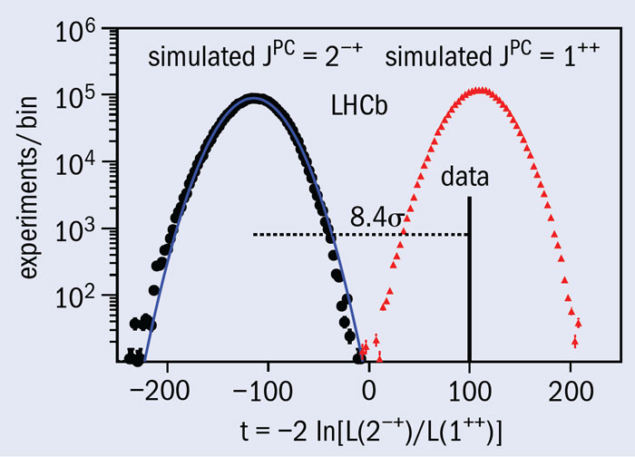# LHCb pins down X(3872) quantum numbers

20 February 2013

One of the most interesting discoveries of the past decade is that of an unconventional hadron, the X(3872), by the Belle experiment (Belle 2003). Its decay to J/ψπ+π indicates that it is charmonium-like but its narrow width and mass above the threshold for decay to open charm do not fit any of the spectrum of predicted cc states. Several experiments have since confirmed this observation, in different production mechanisms and decay modes. In parallel to these experimental investigations, many theoretical interpretations have been put forward but the fundamental question remains open of whether the X(3872) is a quark–antiquark meson or a more exotic state.Fig. 1. Distribution of the test statistic for simulated experiments with JPC = 1++ (red) or 2–+(blue), compared with the result from a data sample where the X(3872) is produced in B+ decays. The fit clearly prefers JPC = 1++.

When any new resonance is observed it is mandatory to determine its quantum numbers. The observation of the decay X(3872)→J/ψγ fixed the charge conjugation: C = +1. However, angular analyses left two possibilities for JPC: 1++ and 2–+ (CDF 2007). Exotic models where the X(3872) is a DD* molecule or a tetraquark state predict JPC = 1++.

The LHCb collaboration has now reported an analysis of the decay chain B+ → X(3872)K+ → J/ψπ+πK+, with J/ψ → μ+μ, where they use all five angular variables to maximize the separation power between the hypotheses of 1++ and 2–+. The analysis uses the data sample of 1.0 fb–1 that LHCb collected during 2011, which contains 313 ± 26 B+ → X(3872)K+ decays. As figure 1 shows, the outcome of the multidimensional likelihood fit prefers JPC = 1++ with more than 8σ significance. Compared with previous analyses, the measurement benefits from larger statistics but importantly also makes use of the full angular information, which improves the ability to use correlations between angular variables to separate the two hypotheses (figure 2 shows an example).Fig. 2. Distribution of the cosine of the decay angle of the X(3872) for all candidates and for a subset with a selection on the cosine of the decay angle of the π+π– system. The selected shows a clearer separation between the hypotheses, coloured as in figure 1.

This result rules out explanations of the X(3872) as the ηc2(11D2) state. Instead, it favours more exotic interpretations. However, distinguishing between molecular and tetraquark models will require studies of complementary decay modes. The 2.0 fb–1 data sample that LHCb accumulated during 2012, as well as the larger samples that will be recorded in future LHC runs, will allow the collaboration to keep on the trail of these and other puzzles in heavy-flavour spectroscopy.